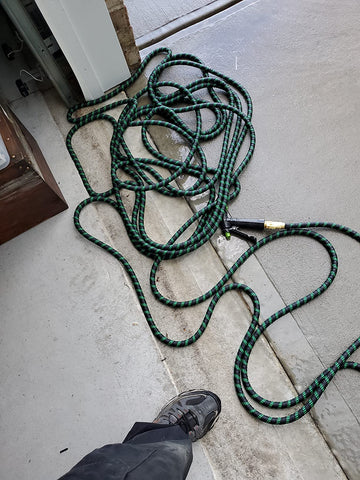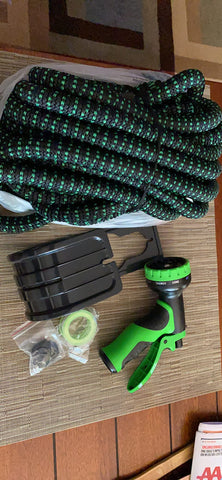The capacity of a 100-foot length of 1-inch diameter garden hose is 0.326 gallons.

Assuming the 100ft garden hose is completely filled with water, the volume of water in the hose can be calculated as follows:First, convert the diameter from inches to feet: 1 inch = 1/12 feet.
Calculate the cross-sectional area of the hose: A = πr^2, where r is the radius of the hose (which is half the diameter). So, r = (1/2)/12 = 1/24 feet. Therefore, A = π(1/24)^2 = 0.000436 square feet.

Multiply the cross-sectional area by the length of the hose to get the volume: V = AL = 0.000436 × 100 = 0.0436 cubic feet.
To convert cubic feet to gallons, we need to know the specific weight of water, which is 62.4 pounds per cubic foot. One gallon of water weighs 8.34 pounds.Convert the volume in cubic feet to gallons: 0.0436 × 7.48 = 0.326 gallons (rounded to three decimal places).
So there are approximately 0.326 gallons of water in a 100-foot, 1-inch diameter garden hose when it is completely filled with water.